Latest Banking jobs   »

# Quantitative Aptitude Quiz For NABARD Grade A 2022- 22nd August

Q1. Ratio of numerical value of rate of interest and time period is 4 : 1. Man invested Rs. 2400 and gets Rs. 864 as simple interest. Find the value of X, if man invested Rs. (2400 + X) at same rate of interest on C.I. for two years and get Rs. 814.08 as interest?
(a) 600 Rs.
(b) 800 Rs.
(c) 400 Rs.
(d) 200 Rs.
(e) 540 Rs.

Q2. Ratio of speed of faster train to slower train is 9 : 7 and length of two trains is 120 m and 180 m respectively. If both trains increased their respective speed by 25% and then cross each other in 36 sec running in same direction, then find in what time both trains cross each other running in opposite direction with their usual speed?
(a) 64/6 sec
(b) 58/6 sec
(c) 52/6 sec
(d) 49/8 sec
(e) 45/8 sec

Q3. A and B entered into a business with the capital of Rs. (X + 1200) and Rs (X+1500) respectively. After eight months from starting A withdraw half of his investment and B doubled his investment. If at the end of the year A got Rs. 4250 as profit share out of total profit of Rs. 11250, then find three times of initial investment of B ?
(a) Rs 32,400
(b) Rs 30,750
(c) Rs 30,500
(d) Rs 31,500
(e) Rs 31,770

Q4. Two farmers A and B have mixture of potassium and Urea in the quantity of (θ + 54) kg & (θ + 84) kg respectively. The ratio of potassium and Urea in mixture which farmer A has is 3 : 2, while farmer B has 2 : 1. If 60% and 66 ⅔% of their respective mixture farmer A and B used for their field and the remaining mixture of A and B are equal, then find the quantity of potassium in the mixture of farmer B has ?
(a) 60 kg
(b) 120 kg
(c) 90 kg
(d) 100 kg
(e) 75 kg

Q5. A bag contains seven red balls, ‘a’ green balls & ‘b’ yellow balls. If one ball taken out from bag, then probability of being it green is 5/16, while being it yellow is ¼. Find difference between number of green and yellow balls in that bag?
(a) 1
(b) 0
(c) 2
(d) 4
(e) 3

Q6. Mohan mixed two types of wheats costing Rs. 80 per kg and Rs. 120 per kg in the ratio of 1 : 3 and sold this mixture at the price of Rs. 143 per kg. Find the profit percentage of Mohan?
(a) 25%
(b) 30%
(c) 35%
(d) 40%
(e) 20%

Q7. Gurdeep chhabra joined ‘Adda 247’ with the work experience of 26 years due to which average work experience of all employees of ‘Adda 247’ was increased by one year. If initial average work experience of all employees of ‘Adda 247’ was five years, then find the new number of employees in ‘Adda 247’?
(a) 23
(b) 19
(c) 25
(d) 21
(e) 27

Q8. If total cost of fencing a circular plot is Rs. 2816, then what will be cost of fencing a square plot, which has side of 6.4 meters more than radius of given circular plot having area of 98. 56 m2. (consider per meter cost of fencing of circular plot & of square plot same)
(a) 3860 Rs.
(b) 3820 Rs.
(c) 3840 Rs.
(d) 3800 Rs.
(e) 3880 Rs.

Q9. Ram and Shyam invest in the ratio of 3 : 4 in a partnership business and Ram got Rs. 2700 as profit share out of total profit of Rs. 5100, then find the ratio of period of investment of Shyam to that of Ram?
(a) 3 : 4
(b) 3 : 5
(c) 2 : 3
(d) 1 : 2
(e) 3 : 2

Q10. A wooden seller has some number of tables which are 25% more than number of chairs. 60% of tables and 50% of chairs are sold, each table is at Rs. 250 and each chair is at Rs. 240. If number of sold tables are 30 more than that of sold chair, then find difference between total selling price of sold tables and sold chairs (in Rs.)?
(a) 8200
(b) 8100
(c) 9100
(d) 8400
(e) 8500

Q11. When we reverse the digits of a two-digit number, we got a new number. Product of new number with sum of its digits is 52 and digit at ten’s place of the original number is 2 more than the digit at unit’s place of original number. Find original number?
(a) 39
(b) 33
(c) 41
(d) 31
(e) 37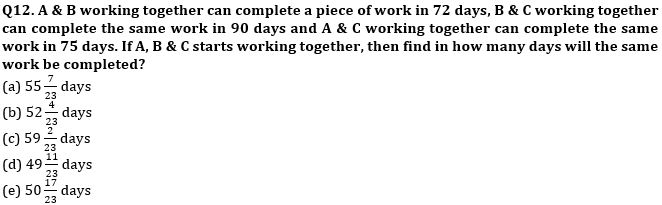Q13. Cost price of an article is 39% less than the marked price of the article and shopkeeper earned 40% profit in selling the article. If amount of profit is Rs.196 more than amount of discount, then find cost price of article.
(a) Rs.1345
(b) Rs.1325
(c) Rs.1290
(d) Rs.1245
(e) Rs.1220

Q14. Time taken by boat to cover 48 km in upstream is 200% of the time taken by boat to cover 48 km in downstream. If sum of time taken by boat to cover 48 km distance in upstream and same distance in downstream is 9 hours, then find speed of boat?
(a) 8 km/hr
(b) 10 km/hr
(c) 12 km/hr
(d) 4 km/hr
(e) 6 km/hr

Q15. Pankaj and Suresh invested capital in the ratio of 4 : 3 in a partnership business. Pankaj and Suresh left the business after 5 months and after 10 months respectively. Raju joined the business with Rs.50000 when Suresh left, and they wind up the business after 1 year. If at the end of one-year profit sharing ratio of Pankaj, Suresh & Raju is 2 : 3 : 1, then find the difference between the capital invested by Pankaj and Suresh?
(a) Rs.10000
(b) Rs.40000
(c) Rs.30000
(d) Rs.50000
(e) Rs.20000

Solutions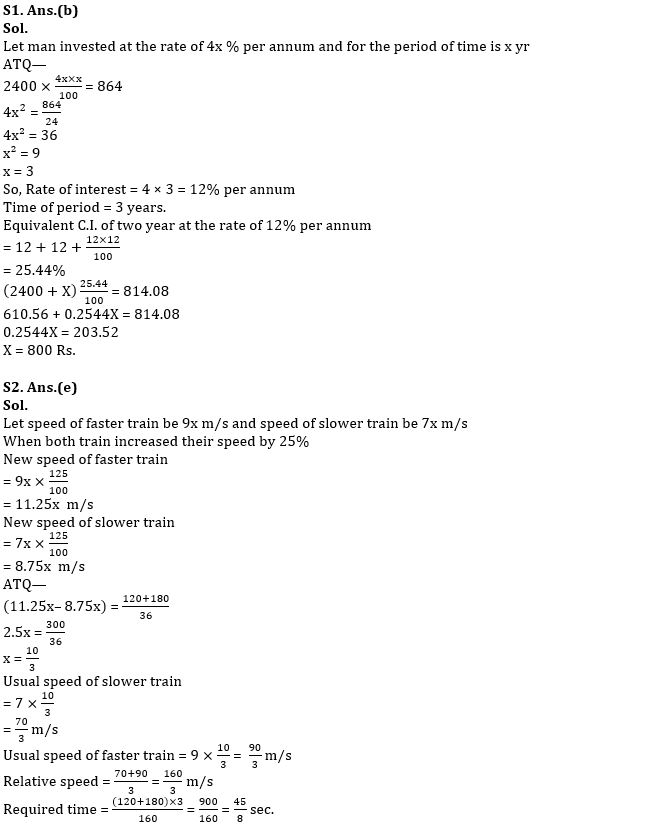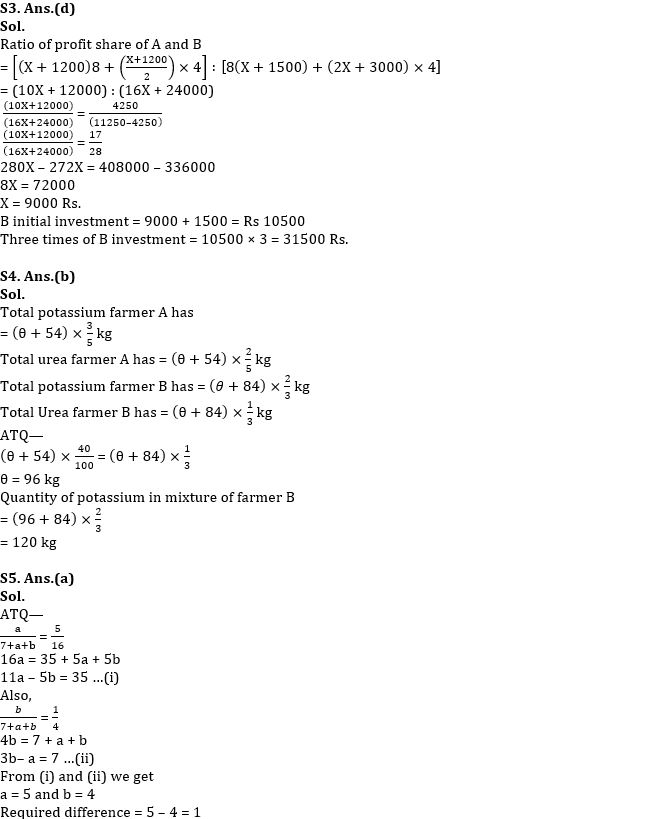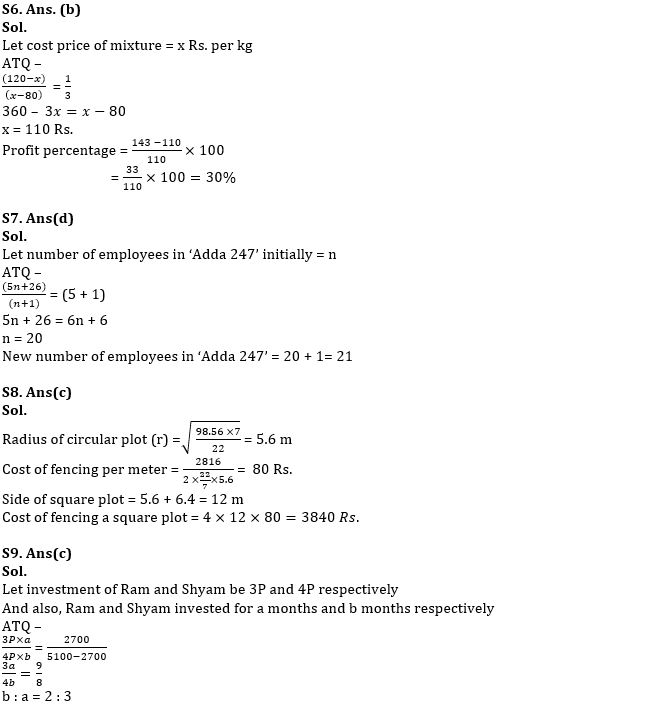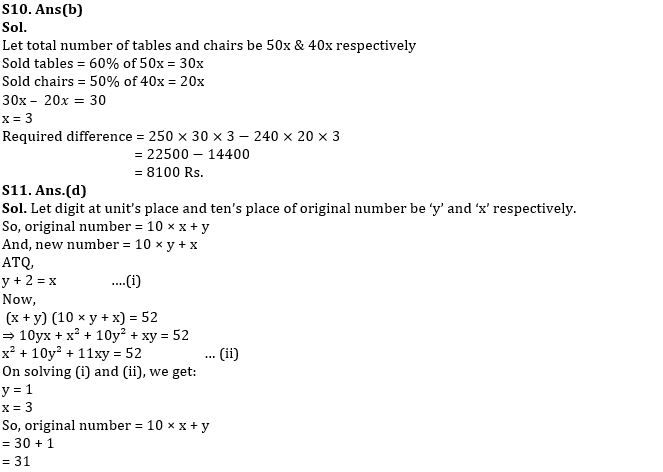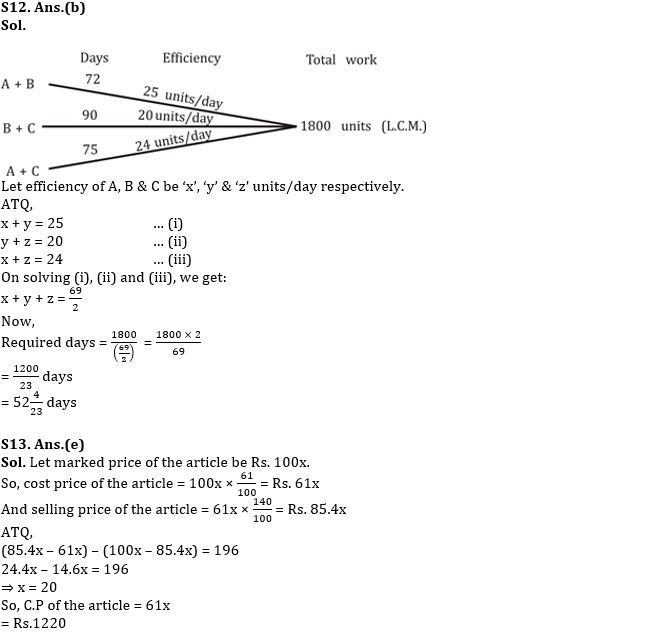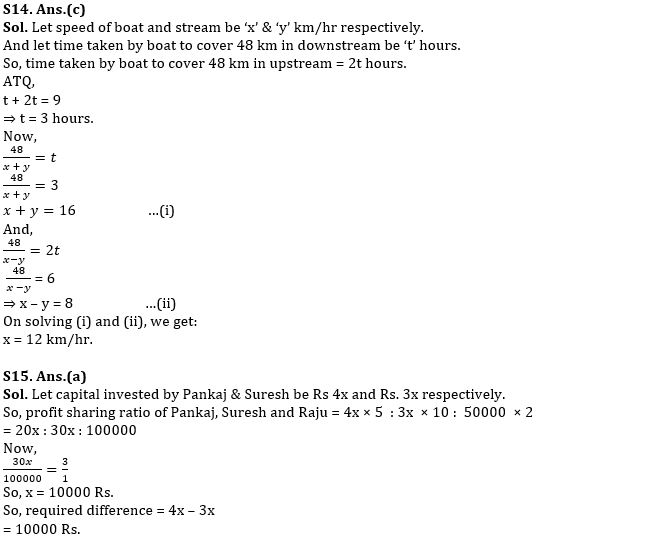#### Congratulations!Download Hindu Review of October 2021: Free PDFIncorrect details? Fill the form again here

Download Hindu Review of October 2021: Free PDF

Thank You, Your details have been submitted we will get back to you.
•General Awareness Quiz Series 2022: 4th ...
•World Animal Welfare Day 2022, Theme, Hi...
•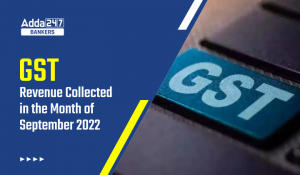GST revenue collected in the month of Se...
•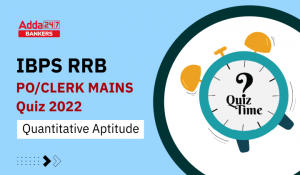Quantitative Aptitude Quiz For IBPS RRB ...
•American Express ties up with Nasscom to...
•Active credit cards drop 2.3 million in ...